GRE Subject Test: Chemistry : Reactions with Inorganic Compounds

Example Questions

Example Question #11 : Reaction Chemistry

What happens when a solution of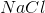is added to a solution of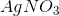?

A combustion reaction

A decomposition reaction

A double displacement reaction

A combination reaction

A synthesis reaction

A double displacement reaction

Explanation:

The chemical reaction that would occur is provided below: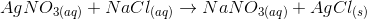This type of reaction is called a double displacement reaction in which there is an exchange of ions between two compounds. This type of reaction usually results in the formation of a precipitate or gas. In terms of the positive ions, the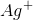switch places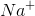to form two new products.

Example Question #1 : Reactions With Inorganic Compounds

Which of the following types of reactions best describes the following reaction: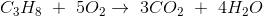Alkylation

Catalytic

Synthesis

Combustion

Combustion

Explanation:

The type of reaction given is termed a combustion reaction. A combustion reaction is one that involves a substance reacting with molecular oxygen. For combustion reactions involving a hydrocarbon as given in the example, the major products are carbon dioxide and water. The word combustion is a synonym for burn.

Example Question #2 : Reactions With Inorganic Compounds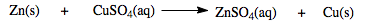For the reaction given, identify the type of reaction taking place.

Displacement reaction

Decomposition reaction

Combination reaction

Synthesis reaction

Displacement reaction

Explanation:

A displacement reaction is one in which an atom, ion or molecule moves from one compound to another to replace an atom, ion or molecule in another compound. In the reaction given,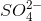ion moves from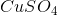to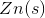to form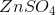.

Example Question #1 : Reactions With Inorganic Compounds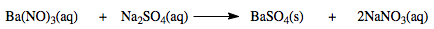Determine the percentage yield of the reaction given if 12.6 grams of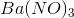is reacted excess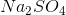to give 10.4 grams of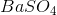.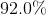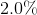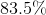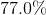Explanation:

In chemistry, percentage yield is a term used to quantify the efficiency of a chemical reaction. In order to calculate this quantity, the value for the actual yield and theoretical yield is needed. The actual yield is the amount of product obtained during a chemical reaction. The theoretical yield is the maximum amount of product possible to be obtain by a chemical reaction which is based on the amount of reactants used. Below is the formula needed to calculate the percentage yield.

Convert the number of grams of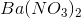to moles: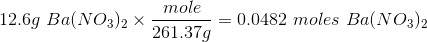Based on the chemical equation 1 mole ofis produced for every 1 mole ofreacted.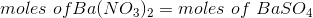Convert the moles ofto grams: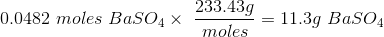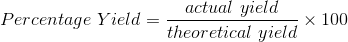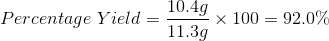Example Question #151 : Gre Subject Test: Chemistry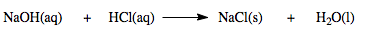Determine the percentage yield of the reaction given if 21.0 grams of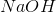is reacted with excess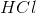to give 10.2 grams of.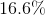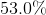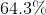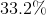Explanation:

In chemistry, percentage yield is a term used to quantify the efficiency of a chemical reaction. In order to calculate this quantity, the value for the actual yield and theoretical yield is needed. The actual yield is the amount of product obtained during a chemical reaction. The theoretical yield is the maximum amount of product possible to be obtain by a chemical reaction which is based on the amount of reactants used. Below is the formula needed to calculate the percentage yield.

Convert the number of grams ofto moles: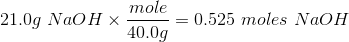Based on the chemical equation 1 mole ofis produced for every 1 mole ofreacted.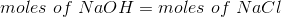Convert the moles ofto grams: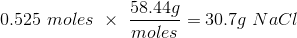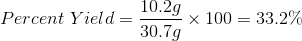Example Question #2 : Reactions With Inorganic Compounds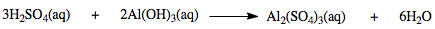Determine the theoretical yield in grams of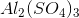produced if 5.6 grams of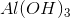is reacted with excess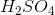.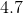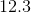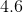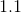Explanation:

Convert the number of grams ofto moles: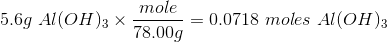Based on the chemical equation 1 mole ofis produced for every 2 mole ofreacted.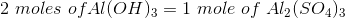Therefore: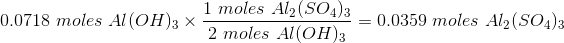Convert the moles ofto grams: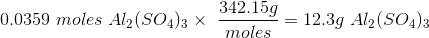Example Question #5 : Reactions With Inorganic Compounds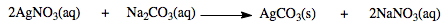For the reaction given, identify the type of reaction taking place.

Combination reaction

Synthesis reaction

Decomposition reaction

Double displacement reaction

Double displacement reaction

Explanation:

A displacement reaction is one in which an atom, ion or molecule moves from one compound to another to replace an atom, ion or molecule in another compound. In the reaction given,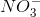ion moves fromto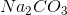. Also,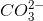ion moves fromto.

Example Question #6 : Reactions With Inorganic Compounds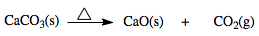Determine the percentage yield of the reaction given if 40.0 grams of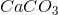is heated to give 15.0 grams of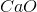.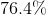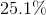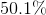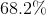Explanation:

In chemistry, percentage yield is a term used to quantify the efficiency of a chemical reaction. In order to calculate this quantity, the value for the actual yield and theoretical yield is needed. The actual yield is the amount of product obtained during a chemical reaction. The theoretical yield is the maximum amount of product possible to be obtain by a chemical reaction which is based on the amount of reactants used. Below is the formula needed to calculate the percentage yield.

Convert the number of grams ofto moles: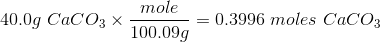Based on the chemical equation 1 mole ofis produced for every 1 mole ofreacted.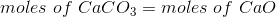Convert the moles ofto grams: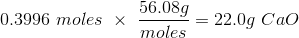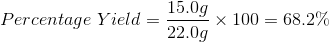Example Question #2 : Reactions With Inorganic Compounds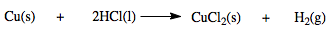For the reaction given, identify the type of reaction taking place.

Displacement reaction

Decomposition reaction

Combination reaction

Synthesis reaction

A displacement reaction is one in which an atom, ion or molecule moves from one compound to another to replace an atom, ion or molecule in another compound. In the reaction given, 2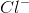ions move from 2 moles ofto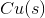to form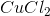.